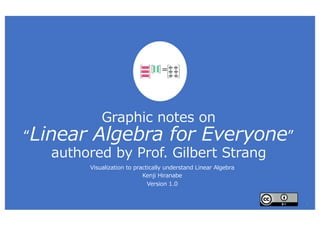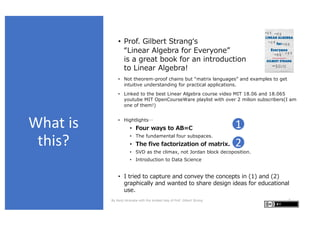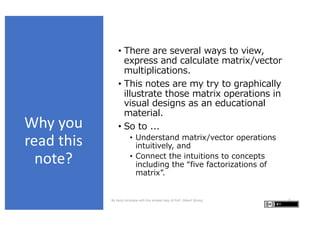Successfully reported this slideshow.

# Graphic Notes on Introduction to Linear Algebra

0

Share×
1 of 19
1 of 19

# Graphic Notes on Introduction to Linear Algebra

0

Share

Graphic Notes on Introduction to Linear Algebra authored by Prof. Gilbert Strang.

This is an idea for visualization to better understand linear algebra.
If you want a PowerPoint version, feel free to let me know, I'll share it with you.

Graphic Notes on Introduction to Linear Algebra authored by Prof. Gilbert Strang.

This is an idea for visualization to better understand linear algebra.
If you want a PowerPoint version, feel free to let me know, I'll share it with you.

## More Related Content

### Related Books

Free with a 30 day trial from Scribd

See all

### Related Audiobooks

Free with a 30 day trial from Scribd

See all

### Graphic Notes on Introduction to Linear Algebra

1. 1. Graphic notes on “Linear Algebra for Everyone” authored by Prof. Gilbert Strang Visualization to practically understand Linear Algebra Kenji Hiranabe Version 1.0 = 1
2. 2. What is this? • Prof. Gilbert Strangʻs “Linear Algebra for Everyone” is a great book for an introduction to Linear Algebra! • Not theorem-proof chains but “matrix languages” and examples to get intuitive understanding for practical applications. • Linked to the best Linear Algebra course video MIT 18.06 and 18.065 youtube MIT OpenCourseWare playlist with over 2 milion subscribers(I am one of them!) • Hightlights… • Four ways to AB=C • The fundamental four subspaces. • The five factorization of matrix. • SVD as the climax, not Jordan block decoposition. • Introduction to Data Science • I tried to capture and convey the concepts in (1) and (2) graphically and wanted to share design ideas for educational use. By Kenji Hiranabe with the kindest help of Prof. Gilbert Strang 2 1 2
3. 3. Why you read this note? • There are several ways to view, express and calculate matrix/vector multiplications. • This notes are my try to graphically illustrate those matrix operations in visual designs as an educational material. • So to ... • Understand matrix/vector operations intuitively, and • Connect the intuitions to concepts including the “five factorizations of matrix”. By Kenji Hiranabe with the kindest help of Prof. Gilbert Strang 3
4. 4. Table of Contents • Viewing a Matrix – 4 Ways • Vector times Vector • Matrix times Vector – 2 Ways • Matrix times Matrix – 4 Ways • Practical Patterns • The Five Matrix Factorizations • 𝐶𝑅, 𝐿𝑈, 𝑄𝑅, 𝑄Λ𝑄!, 𝑈Σ𝑉! By Kenji Hiranabe with the kindest help of Prof. Gilbert Strang 4
5. 5. = = = 2 column vectors with 2 numbers 3 row vectors with 2 numbers 6 Numbers1 Matrix Viewing a Matrix – Four Ways 𝐴 = 𝑎!! 𝑎!" 𝑎"! 𝑎"" 𝑎#! 𝑎#" = | 𝒂 𝟏 | | 𝒂 𝟐 | = −𝒂! ∗ − −𝒂" ∗ − −𝒂# ∗ − Here, column vectors are in bold as 𝒂 𝟏, row vectors are with * as 𝒂" ∗ . And transposed vectors/matrices are with T on the shoulders as 𝒂 𝑻, 𝑨 𝑻 𝐴 = 1 4 2 5 3 6 = 1 4 2 5 3 6 = 1 4 2 5 3 6 By Kenji Hiranabe with the kindest help of Prof. Gilbert Strang 5
6. 6. = = =Dot product(number) Rank1 Matrix v1 = 1 2 3 𝑥 𝑦 = 𝑥 𝑦 2𝑥 2𝑦 3𝑥 3𝑦 Vector times Vector 1 2 3 𝑥! 𝑥" 𝑥# = 1 2 3 , 𝑥! 𝑥" 𝑥# = 𝑥! + 2𝑥" + 3𝑥# 𝑎𝑏! is a Matrix (𝑎𝑏! = 𝐴). If neither 𝑎, 𝑏 are 0 The result 𝐴 is a rank1 Matrix. Dot product (𝑎 ) 𝑏) is expressed as 𝑎! 𝑏 in matrix language and yields a number. v2 By Kenji Hiranabe with the kindest help of Prof. Gilbert Strang 6
7. 7. = = + Matrix times Vector – 2 Ways The row vectors of A are multiplied by a vector x and become the three dot-product elements of the produced vector. The produced vector is a linear combination of the column vectors of A. 𝑨𝒙 = 1 2 3 4 5 6 𝑥! 𝑥" = (𝑥!+2𝑥") (3𝑥! + 4𝑥") (5𝑥! + 6𝑥") 𝑨𝒙 = 1 2 3 4 5 6 𝑥! 𝑥" = 𝑥! 1 3 5 + 𝑥" 2 4 6 At first, you learn (Mv1). But when you get used to viewing it as (Mv2), you can understand 𝑨𝒙 as a linear combination of the columns of A, which spans the column space of A denoted as C 𝑨 , and further, see the solution space of 𝑨𝒙 = 𝟎 as the nullspace of A denoted as N 𝑨 . Mv1 Mv2 By Kenji Hiranabe with the kindest help of Prof. Gilbert Strang 7
8. 8. Matrix times Matrix – 4 Ways = = + 1 2 3 4 5 6 𝑥" 𝑦" 𝑥# 𝑦# = (𝑥"+2𝑥#) (𝑦"+2𝑦#) (3𝑥"+4𝑥#) (3𝑦"+4𝑦#) (5𝑥"+6𝑥#) (5𝑦"+6𝑦#) 1 2 3 4 5 6 𝑥" 𝑦" 𝑥# 𝑦# = 𝑨 𝒙 𝒚 = 𝑨 𝒙 𝑨𝒚 = = = = 1 2 3 4 5 6 𝑏"" 𝑏"# 𝑏#" 𝑏## = 𝒂 𝟏 𝒂 𝟐 𝒃 𝟏 ∗ 𝒃 𝟐 ∗ = 𝒂 𝟏 𝒃 𝟏 ∗ + 𝒂 𝟐 𝒃 𝟐 ∗ = 1 3 5 𝑏"" 𝑏"# + 2 4 6 𝑏#" 𝑏## = 𝑏"" 𝑏"# 3𝑏"" 3𝑏"# 5𝑏"" 5𝑏"# + 2𝑏#" 2𝑏## 4𝑏#" 4𝑏## 6𝑏#" 6𝑏## The produced columns 𝑨𝒙, 𝑨𝒚 are linear combinations of columns of A. 1 2 3 4 5 6 𝑥" 𝑦" 𝑥# 𝑦# = 𝒂 𝟏 ∗ 𝒂 𝟐 ∗ 𝒂 𝟑 ∗ 𝑿 = 𝒂 𝟏 ∗ 𝑿 𝒂 𝟐 ∗ 𝑿 𝒂 𝟑 ∗ 𝑿 Multiplication of a Matrix is broken down to sum of Rank1 matrices. The produced rows are linear combinations of rows. Every elements becomes a dot product of row vector and column vector. MM 1 MM 2 MM 3 MM 4 By Kenji Hiranabe with the kindest help of Prof. Gilbert Strang 8
9. 9. Practical Patterns (1/3) 2 3 = 1 2 31 1 = 21 + 3 + 2 = 21 + 3 + 3 = 21 + 3 + 1 2 3 = 1 2 3 1 = +1 2 3+ 2 = +1 2 3+ 3 = +1 2 3+ MM 2 Mv2 MM 3 Operations from the right effect to the columns of the Matrix. This expression can be seen as the three linear combinations in the right in one formula. P1 P2 using using By Kenji Hiranabe with the kindest help of Prof. Gilbert Strang 9 Operations from the left effect to the rows of the Matrix. This expression can be seen as the three linear combinations in the right in one formula.
10. 10. = = 𝐴𝐷 = 𝒂 𝟏 𝒂 𝟐 𝒂 𝟑 𝑑! 𝑑" 𝑑# = 𝑑! 𝒂 𝟏 𝑑" 𝒂 𝟐 𝑑" 𝒂 𝟐 𝐷𝐵 = 𝑑! 𝑑" 𝑑# 𝒃! ∗ 𝒃! ∗ 𝒃! ∗ = 𝑑! 𝒃! ∗ 𝑑! 𝒃! ∗ 𝑑! 𝒃! ∗ Applying a diagonal matrix from the right scales each column. Applying a diagonal matrix from the left scales each row. Burn this into your memories and you can see … P1’ P2’ By Kenji Hiranabe with the kindest help of Prof. Gilbert Strang 10 Practical Patterns (2/3)
11. 11. Practical Patterns (3/3) = + + A double combination of columns. You will encounter this in differential/recurrence equations. = 𝑿𝑫𝒄 = 𝒙 𝟏 𝒙 𝟐 𝒙 𝟑 𝑑! 𝑑" 𝑑# 𝑐! 𝑐" 𝑐# = 𝑐! 𝑑! 𝒙 𝟏 + 𝑐" 𝑑" 𝒙 𝟐+ 𝑐# 𝑑# 𝒙 𝟑 𝑼𝚺𝑽 𝑻 = 𝒖 𝟏 𝒖 𝟐 𝒖 𝟑 𝜎! 𝜎" 𝜎# 𝒗! - 𝒗" - 𝒗# - = 𝜎! 𝒖! 𝒗! - + 𝜎" 𝒖" 𝒗" - + 𝜎# 𝒖# 𝒗# - + + A matrix is broken down to sum of rank1 matrices, as in singular value/spectrum decomposition. P3 P4 By Kenji Hiranabe with the kindest help of Prof. Gilbert Strang 11
12. 12. The Five Matrix Factorization 𝐴 = 𝐶𝑅 𝐴 = 𝐿𝑈 𝐴 = 𝑄𝑅 𝑆 = 𝑄Λ𝑄! 𝐴 = 𝑈Σ𝑉! By Kenji Hiranabe with the kindest help of Prof. Gilbert Strang 12 Independent column vectors times row echelon form to show row rank = column rank LU decomposition as Gaussian elimination QR decomposition as Gram-Schmidt orthogonalization Eigenvalue decomposition symmetric matrix Singular value decomposition of all matrices
13. 13. 𝐴 = 𝐶𝑅 Procedure: Looking at each A ‘s column vector from left to right. Keep independent ones, discard dependent ones which can be created by the former columns. The col-1,2 survive, and the col-3 is discarded because it is expressed as col-1 + col-2. To rebuilding A by the independent columns 1, 2, you find a row echelon form R. appears in the right. Any general rectangular matrices A has the same row rank as the column rank. This factorization is the most intuitive way to understand this theorem. C consists of independent columns of A. And R is a row reduced echelon form of A. 2 = 1 2 3 2 3 5 = 1 2 2 3 1 0 1 0 1 1 1 | 𝒂 𝟏 | | 𝒂 𝟐 | | 𝒂 𝟑 | = | 𝒄 𝟏 | | 𝒄 𝟐 | 1 0 1 0 1 1 𝒂 𝟏 = 𝒄 𝟏, 𝒂 𝟐= 𝒄 𝟐, 𝒂 𝟑 = 𝒄 𝟏 + 𝒄 𝟐 −𝒂" ∗ − −𝒂# ∗ − = 1 2 2 3 −𝒓" ∗ − −𝒓# ∗ − 𝒂" ∗ = 𝒓" ∗ + 2𝒓# ∗ , 𝒂# ∗ = 2𝒓" ∗ + 3𝒓# ∗ 213 = 1 + 2 1 + 2 1 + 2 All column vectors of A in the left are linear combinations of c1 and c2.. Meaning that the column rank = dim C(A)=2. = +1 2 1 2 1 2+ = All row vectors of A in the left are linear combinations of r1,r2. Meaning that the row rank =dim C(AT)=2. 𝑨 = 𝑪𝑹 P1 P2 using using By Kenji Hiranabe with the kindest help of Prof. Gilbert Strang 13 𝑨 𝑪 𝑹 𝑨 𝑪 𝑹
14. 14. == + + 𝑨 = | 𝒍 𝟏 | −𝒖! ∗ − + 𝟎 𝟎 𝟎 𝟎 𝟎 𝑨 𝟐 ＝ | 𝒍 𝟏 | −𝒖! ∗ − + | 𝒍 𝟐 | −𝒖" ∗ − + 𝟎 𝟎 𝟎 𝟎 𝟎 𝟎 𝟎 𝟎 𝑨 𝟑 = 𝑳𝑼 𝐴 = 𝐿𝑈 Peel the rank 1 matrix made of the row1 and col1 of A and let the remaining A1 . Do this recursively and decompose A into the sum of rank1 matrices. = + + The other way is to rebuild A is easy. MM 4 Gaussian elimination. Usually, you apply elementary row operation matrices from the left. L is their inverse. 𝐿 𝑈 using By Kenji Hiranabe with the kindest help of Prof. Gilbert Strang 14 𝐴
15. 15. == 𝐴 = 𝑄𝑅 A’s column vectors form a basis and can be adjusted into an orthonormal set of vector s Q. Each column vector of A can be rebuilt from Q and an upper triangular matrix R Gram-Schmidt orthogonalization of a basis. 2 31 | 𝒂 𝟏 | | 𝒂 𝟐 | | 𝒂 𝟑 | = | 𝒒 𝟏 | | 𝒒 𝟐 | | 𝒒 𝟑 | 𝑟"" 𝑟"# 𝑟"( 𝑟## 𝑟#( 𝑟(( 31 + 1 2 + 1 2 + 𝒂 𝟏 = 𝑟"" 𝒒 𝟏 𝒂 𝟐 = 𝑟"# 𝒒 𝟏 + 𝑟## 𝒒 𝟐 𝒂 𝟐 = 𝑟"( 𝒒 𝟏 + 𝑟#( 𝒒 𝟐 + 𝑟(( 𝒒 𝟑 𝑨 = 𝑸𝑹 P1 using By Kenji Hiranabe with the kindest help of Prof. Gilbert Strang 15 𝑄 𝑅𝐴 𝒂 𝟏 𝒂 𝟐 𝒂 𝟑
16. 16. 𝑺 = 𝑸𝜦𝑸-= | 𝒒 𝟏 | | 𝒒 𝟐 | | 𝒒 𝟑 | 𝜆! 𝜆" 𝜆# −𝒒! - − −𝒒" - − −𝒒# - − =𝜆! | 𝒒 𝟏 | −𝒒! - − + 𝜆" | 𝒒 𝟐 | −𝒒" - − + 𝜆# | 𝒒 𝟑 | −𝒒# - − 𝑆 = 𝑄𝛬𝑄3 A symmetric matrix S is diagonalized into Λ by an orthogonal matrix Q and its transpose. And it is broken down into a sum of rank1 projection matrices(known as the spectrum theory). = 𝜆! 𝑷! + 𝜆" 𝑷 𝟐 + 𝜆# 𝑷 𝟑 Eigenvalue decomposition of a symmetric matrix S. All the eigenvalues are real, and all the eigenvectors can be chosen orthonormal. 2 31 = + += 1 2 3 1 1 2 2 3 3 𝑺 = 𝑺 𝑻, 𝑸 𝑻 = 𝑸.𝟏 𝑷 𝟏 𝟐 = 𝑷 𝟐 𝟐 = 𝑷 𝟏 𝟐 = 𝑰 𝑷 𝟏 𝑷 𝟐 = 𝑷 𝟐 𝑷 𝟑 = 𝑷 𝟑 𝑷 𝟏 = 𝑶 P4 using By Kenji Hiranabe with the kindest help of Prof. Gilbert Strang 16 𝑄 𝛬𝐴 𝑄- 𝜆! 𝒒 𝟏 𝒒! - 𝜆" 𝒒 𝟐 𝒒" - 𝜆# 𝒒 𝟑 𝒒# -
17. 17. 𝐴 = 𝑈Σ𝑉-= | 𝒖 𝟏 | | 𝒖 𝟐 | | 𝒖 𝟑 | 𝜎! 𝜎" −𝒗! - − −𝒗" - − =𝜎! | 𝒖 𝟏 | −𝒗! - − + 𝜎" | 𝒖 𝟐 | −𝒗" - − 𝐴 = 𝑈Σ𝑉3 You can find V as an orthonormal basis of ℝ) , and U as an orthonormal basis of ℝ* so that it can be diagonalized into Σ. This is called singular value decomposition. And it is also broken down into a sum of rank1 projection matrices. = 𝜎! 𝒖 𝟏 𝒗! - + 𝜎" 𝒖 𝟐 𝒗" - All matrices including rectangular ones can be singular value decomposed(SVD). = += 1 1 2 2 2 31 1 2 𝑼.𝟏 = 𝑼 𝑻, 𝑽.𝟏 = 𝑽 𝑻 P4 using By Kenji Hiranabe with the kindest help of Prof. Gilbert Strang 17 𝑈 Σ𝐴 𝑉- 𝜎! 𝒖 𝟏 𝒗! - 𝜎" 𝒖 𝟐 𝒗" -
18. 18. References and Credits • Linear Algebra for Everyone http://math.mit.edu/everyone/ • MIT OpenCourseWare 18.06 http://web.mit.edu/18.06/www/vi deos.shtml • A 2020 Vision of Linear Algebra https://ocw.mit.edu/resources/res -18-010-a-2020-vision-of-linear- algebra-spring-2020/ • My blog entry Matrix World https://anagileway.com/2020/09/ 29/matrix-world-in-linear-algebra- for-everyone/ • The four subspaces T-shirt https://anagileway.com/2020/06/ 04/prof-gilbert-strang-linear- algebra/ This work is inspired by Prof. Strangʼs books and lecture videos. I deeply appreciate his work, passion and personality. By Kenji Hiranabe with the kindest help of Prof. Gilbert Strang 18
19. 19. Thank you for reading! Any comments or feedbacks are welcome to: Kenji Hiranabe (hiranabe@gmail.com) = 19